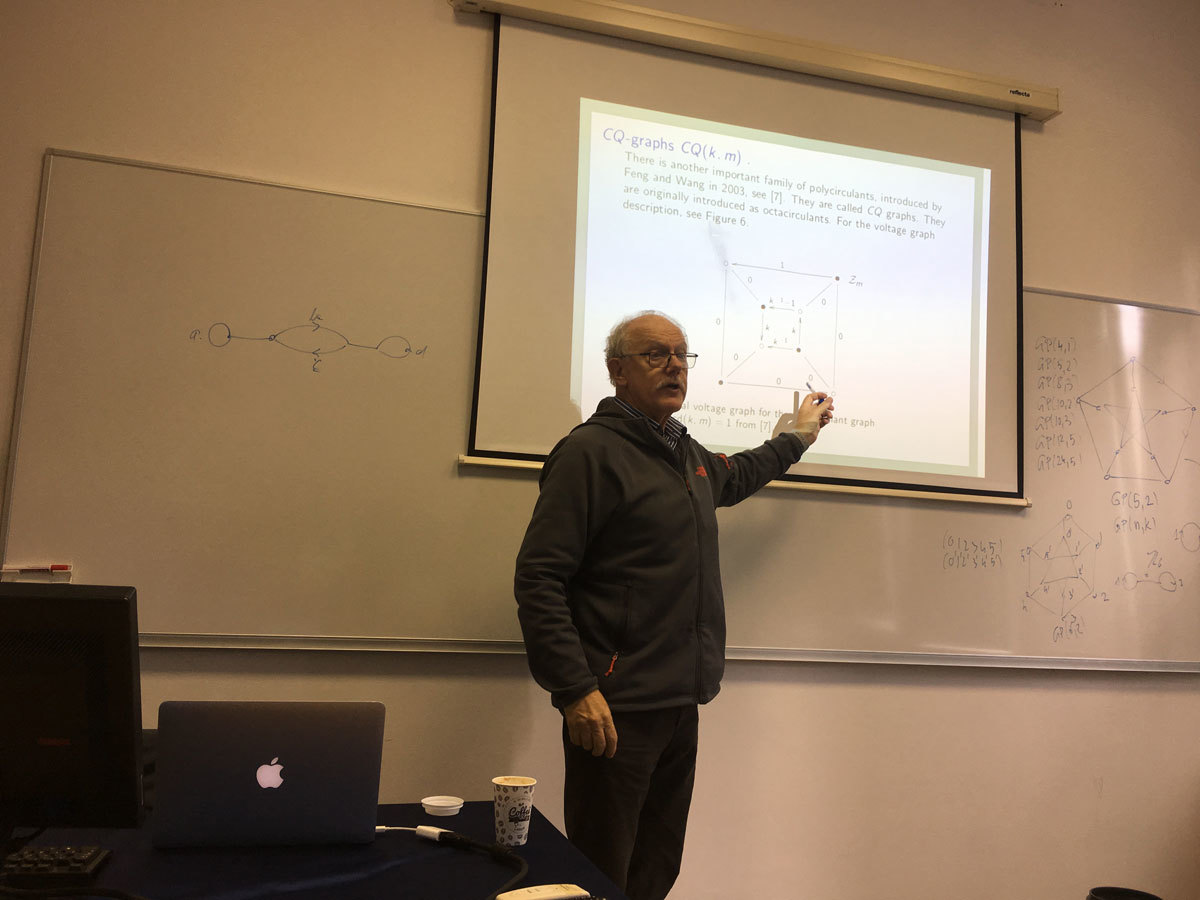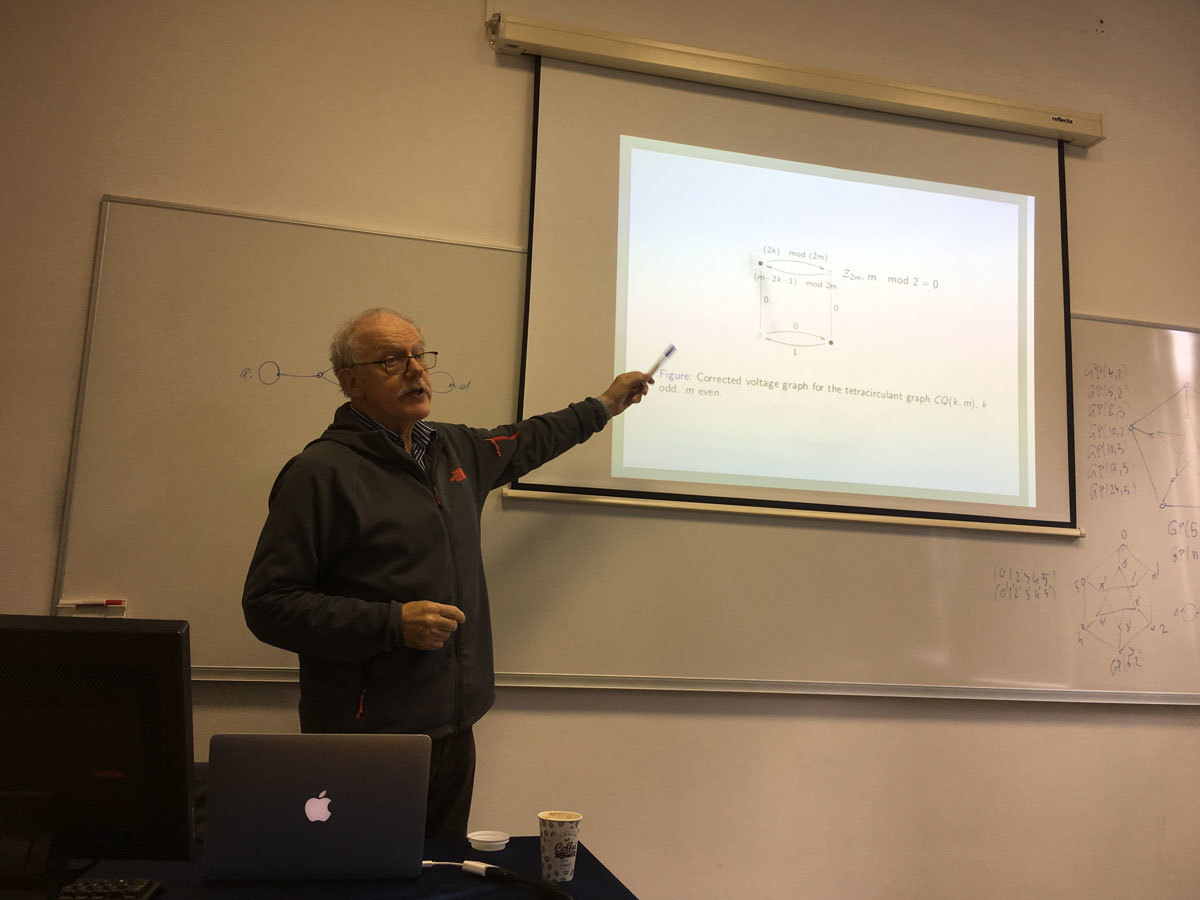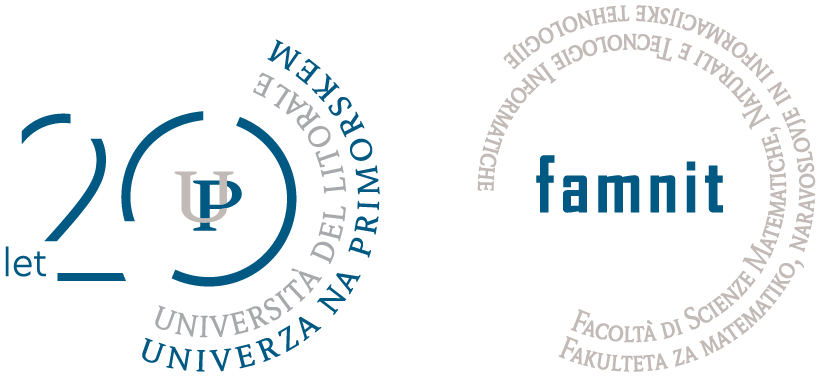Univerza na Primorskem Fakulteta za matematiko, naravoslovje in informacijske tehnologije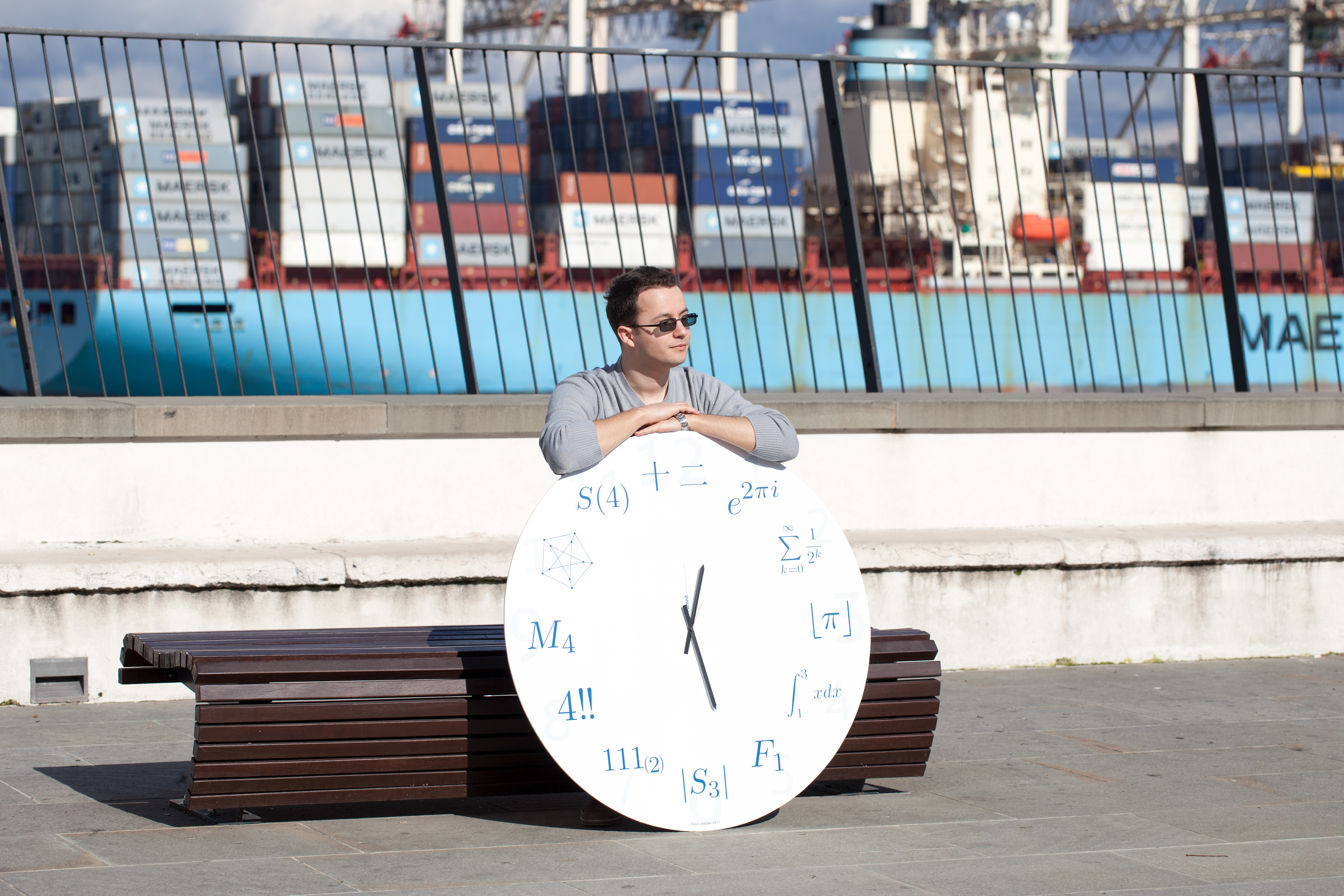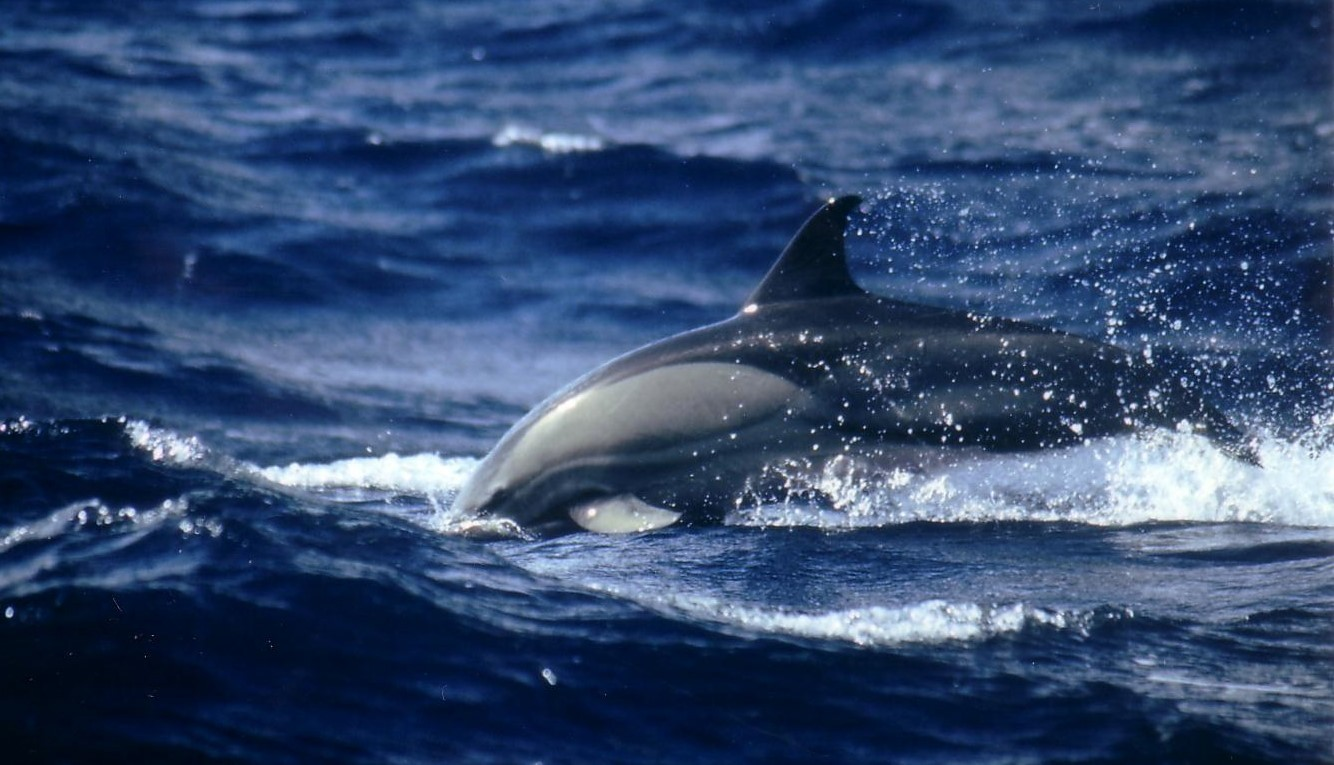SI | EN# Raziskovalni matematični seminar - Arhiv

 2023 2022 2021 2020 2019 2018 2017 2016 2015 2014 2013 2012 2011 2010
 1 2 3 4 5 6 7 8 9 10 11 12
Datum in ura / Date and time: 28.11.18
(10:00-11:00)
Predavalnica / Location: FAMNIT-MP7 (formerly FAMNIT-POŠTA)
Predavatelj / Lecturer: Siamak Yassemi (University of Tehran and Institute for Research in Fundamental Sciences, Iran)
Naslov / Title: Castelnuovo - Mumford regularity of edge ideal of graphs
Vsebina / Abstract:

Let G be a graph with n vertices, S=\mathbb{K}[x_1,\dots,x_n] be the polynomial ring in n variables over a field \mathbb{K} and I(G) denote the edge ideal of G.

We survey a number of recent studies of the Castelnuovo-Mumford regularity of the edge ideal of G. Our focus is on bounds and exact values for the regularity in terms of combinatorial data from associated graphs.

In addition, for every collection \mathcal{H} of connected graphs with K_2\in \mathcal{H}, we introduce the notions of ind-match_{\mathcal{H}}(G) and min-match_{\mathcal{H}}(G). We will improve the inequalities for regularity of S/I(G).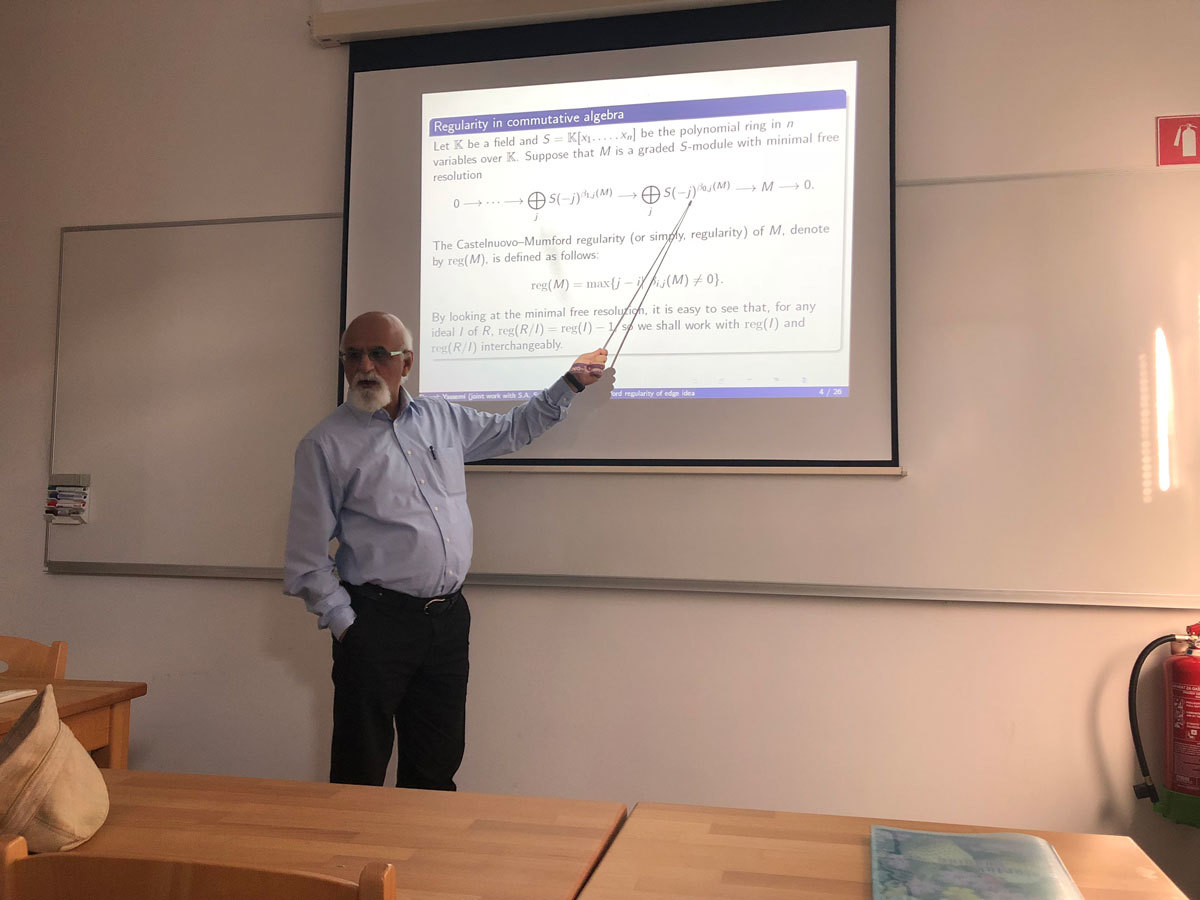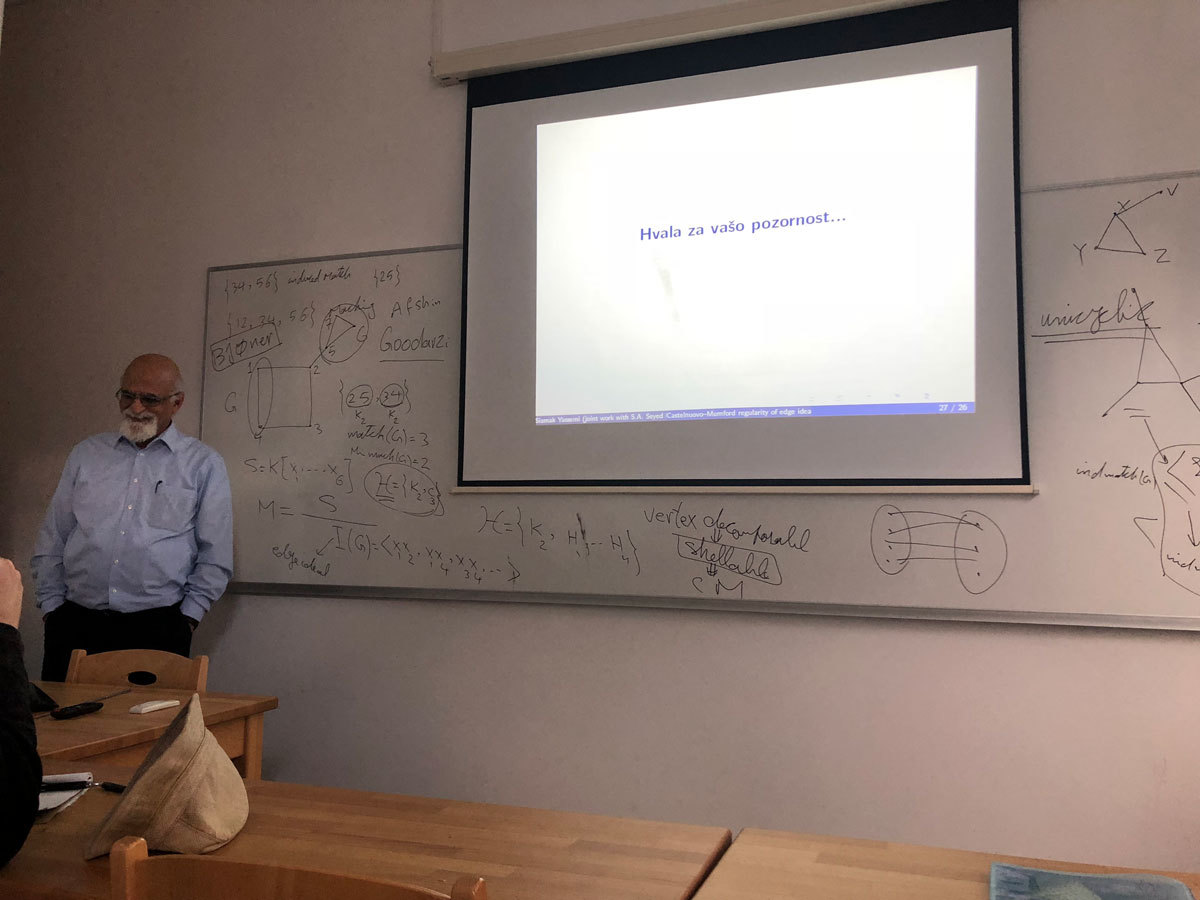Datum in ura / Date and time: 19.11.18
(10:00-11:00)
Predavalnica / Location: FAMNIT-MP7 (formerly FAMNIT-POŠTA)
Predavatelj / Lecturer: Ted Dobson (University of Primorska)
Naslov / Title: On Automorphisms of Haar graphs of Abelian Groups
Vsebina / Abstract:

Let G be a group and S\subseteq G. A Haar graph of G with connection set S has vertex set \Z_2\times G and edge set \{(0,g)(1,gs):g\in G{\rm\ and\ }s\in S\}. Haar graphs are then natural bipartite analogues of Cayley digraphs. We first examine the relationship between the automorphism group of a Cayley digraph of G with connection set S and a Haar graph of G with connection set S. We establish that the automorphism group of a Haar graph contains a natural subgroup isomorphic to the automorphism group of the corresponding Cayley digraph. In the case where G is abelian, we then give four situations in which the automorphism group of the Haar graph can be larger than the natural subgroup corresponding to the automorphism group of the Cayley digraph together with a specific involution, and analyze the full automorphism group in each of these cases. As an application, we show that all s-transitive Cayley graphs of generalized dihedral groups have a quasiprimitive automorphism group, can be reduced" to s-arc-transitive graphs of smaller order, or are Haar graphs of abelian groups whose automorphism groups have a particular permutation group theoretic property.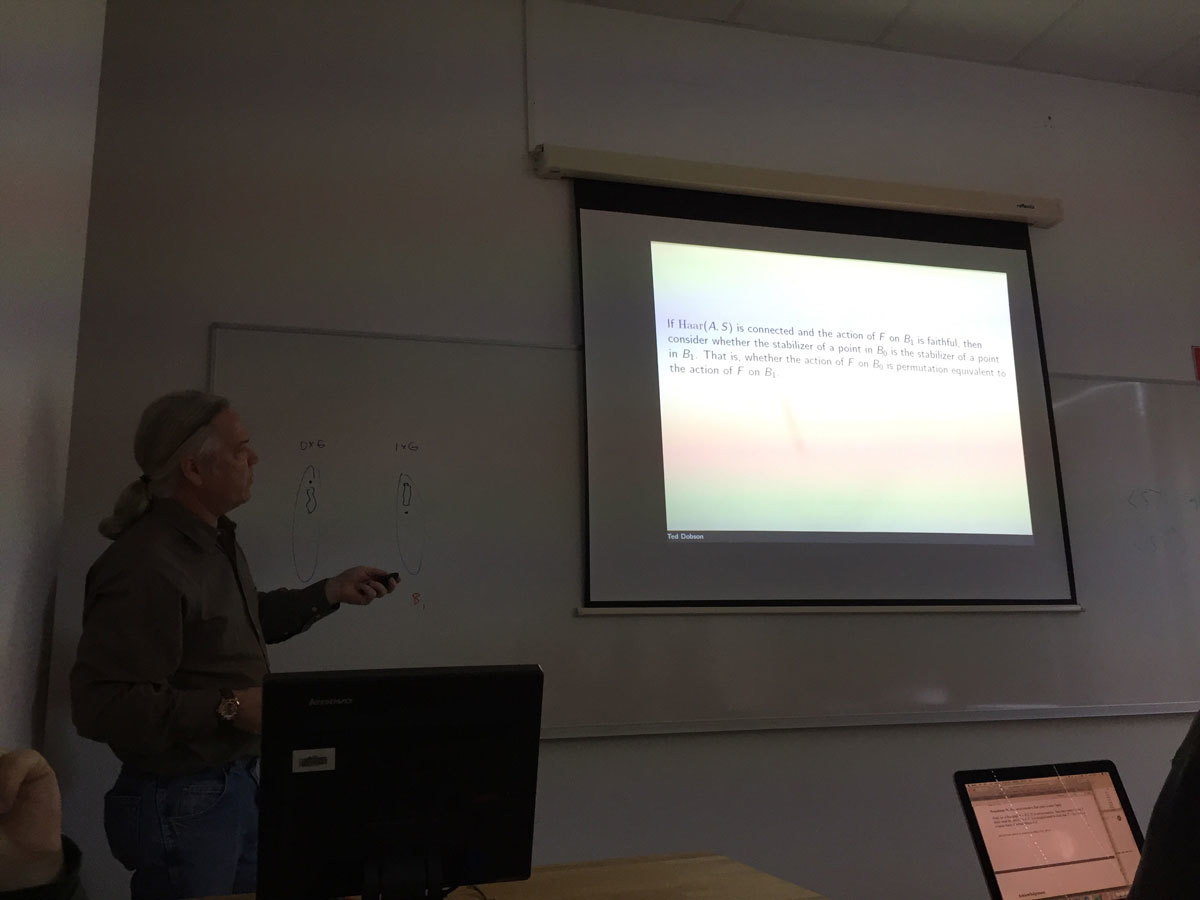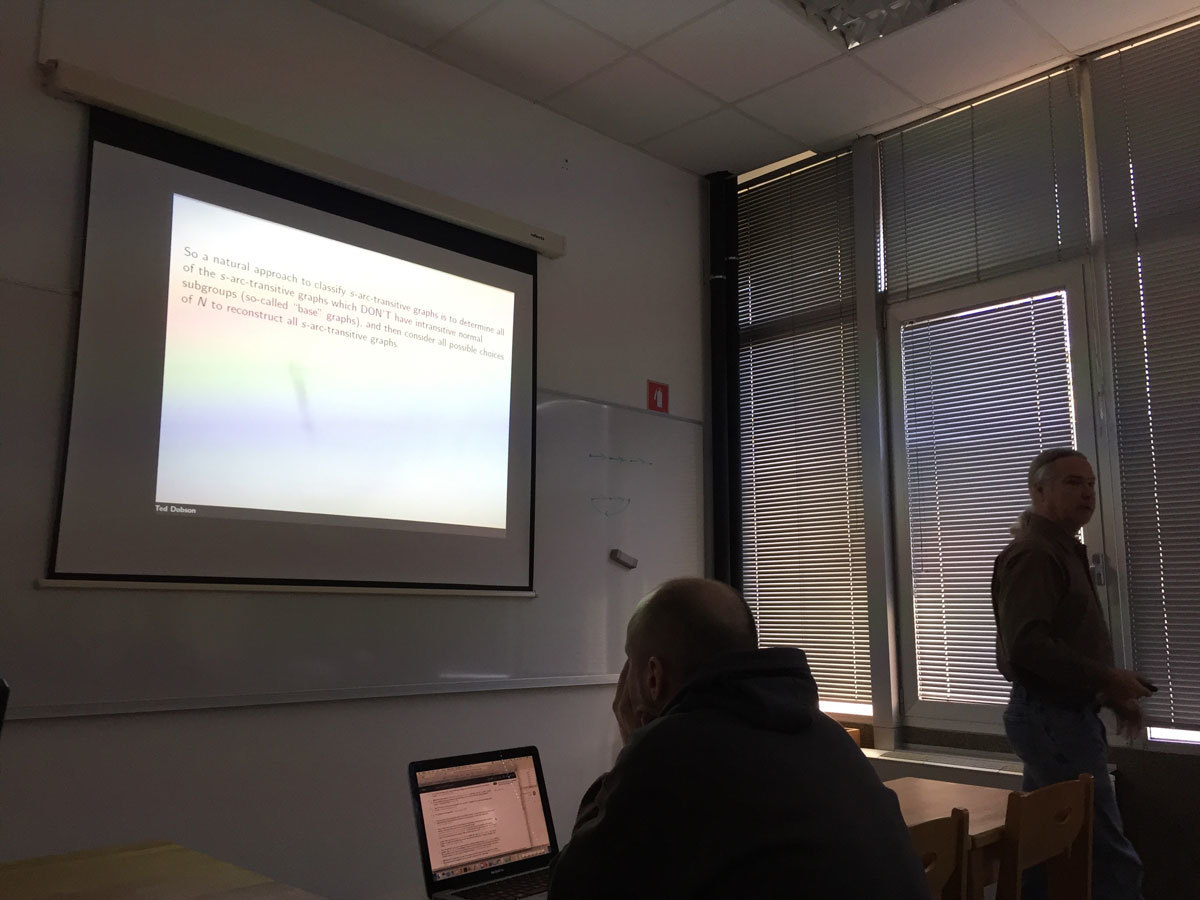Datum in ura / Date and time: 12.11.18
(10:00-11:00)
Predavalnica / Location: FAMNIT-MP7 (formerly FAMNIT-POŠTA)
Predavatelj / Lecturer: Martin Raič (UL FMF and UP FAMNIT)
Naslov / Title: Central limit theorem and the Slepian-Stein method
Vsebina / Abstract:

Roughly speaking, the celebrated central limit theorem says that a sum of many small independent random variables with sufficiently nice distributions approximately follows the normal (Gaussian) distribution. An important issue is the estimation of the error in the normal approximation. Numerous approaches have been suggested. In 1962, Slepian suggested elegant gradual replacement of the original sum with a Gaussian random variable. This approach is essentially equivalent to Stein's method introduced in 1970, which arises from a different idea.

Though relatively old, Stein's method is still a highly active field of research. This is highly due to its flexibility. In particular, Stein's method does not only work for sums of independent random variables, but also for families with various kind of dependence structure. We shall focus on the so called local dependence.Datum in ura / Date and time: 5.11.18
(10:00-11:00)
Predavalnica / Location: FAMNIT-MP7 (formerly FAMNIT-POŠTA)
Predavatelj / Lecturer: Tomaž Pisanski (University of Primorska)
Naslov / Title: A new generalization of generalized Petersen graphs
Vsebina / Abstract:

We discuss a new family of cubic graphs, which we call $SGP$-graphs, that bears a close resemblance to the family of generalized Petersen graphs; both in definition and properties. The focus of our paper is on determining the algebraic properties of graphs from our new family. We look for highly symmetric graphs, e.g., graphs with large automorphism groups, vertex- or arc-transitive graphs. In particular, we present arithmetic conditions for the defining parameters that guarantee that graphs with these parameters are vertex-transitive or Cayley, and we find one arc-transitive $SGP$-graph which is neither a $CQ$ graph of Feng and Wang, nor a generalized Petersen graph.

Joint work with Katarina Jasencakova and Robert Jajcay.